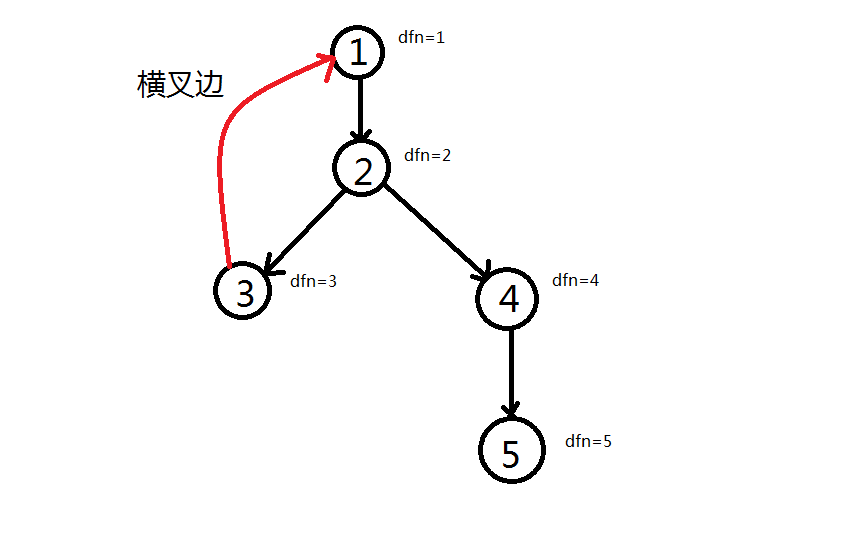# 浅析tarjan算法

Tarjan老爷子一生发明了许多算法下到 NOIP 上到 CTSC 难度的都有。

(Tarjan 算法，并查集，Splay 树，Tarjan 离线求 lca)

# 有向图连通性

## 一些定义

1. 给定有向图$G=(V,E)$，若存在$r\in V$，满足从$r$出发能到达$V$中的所有点，则称$G$为一个“流图”，其中$r$称为流图的源点。

2. 在流图的深度优先遍历中，按照每个节点第一次被访问的顺序，以此给予$N$个节点$1-n$的整数标记，该标记就称为$dfn[x]$，中文名为“时间戳”。

3. 给定一张有向图，若对于图中任意两个节点$x$,$y$，既存在$x$$y$的路径，又存在从$y$$x$的路径，则称该有向图强连通。

4. 强连通分量在有向图G中，如果两个顶点$x$,$y$$（x>y）$有一条从$x$$y$的有向路径，同时还有一条从$x$$y$的有向路径。

1. 在强连通图中各种类型的边，定义边$(x,y)$满足以下条件。

## Tarjan求强连通分量

### 核心思路

#### 初始化### 对应例题

Network of SchoolsPOJ1236

Popular CowsPOJ2186

# 无向图连通性

## 一些定义

1. 若对于$x\in V$，从图中删除$x$与其相邻的所有的边，使$G$分裂为两个或两个以上的子图，则称$x$$G$的割点。

2. 若对于$e\in E$，从图中删除$e$，使$G$分裂为两个或两个以上的子图，则称$e$$G$的割边或桥。

3. 与有向图相似的$dfn$$low$

## Tarjan求割点与桥

### 核心思路

#### 割边判定法则

\begin{aligned} dfn_x

#### 割点判定法则

$x$不是搜索树的根节点，如果$x$是割点当且仅当搜索树上存在$x$的一个子节点$y$。满足：

\begin{aligned} dfn_x\leq low_y \end{aligned}

【模板】割点（割顶）LG3388